Right-click image for copy options.

The image will be full-sized when pasted.

Tip: You can also try dragging the image to your desktop or an application.• Kindergarten
• Number charts
• Skip Counting
• Place Value
• Number Lines
• Subtraction
• Multiplication
• Word Problems
• Comparing Numbers
• Ordering Numbers
• Odd and Even
• Prime and Composite
• Roman Numerals
• Ordinal Numbers
• In and Out Boxes
• Number System Conversions
• More Number Sense Worksheets
• Size Comparison
• Measuring Length
• Metric Unit Conversion
• Customary Unit Conversion
• Temperature
• More Measurement Worksheets
• Writing Checks
• Profit and Loss
• Simple Interest
• Compound Interest
• Tally Marks
• Mean, Median, Mode, Range
• Mean Absolute Deviation
• Stem-and-leaf Plot
• Box-and-whisker Plot
• Permutation and Combination
• Probability
• Venn Diagram
• More Statistics Worksheets
• Shapes - 2D
• Shapes - 3D
• Lines, Rays and Line Segments
• Points, Lines and Planes
• Transformation
• Ordered Pairs
• Midpoint Formula
• Distance Formula
• Parallel, Perpendicular and Intersecting Lines
• Scale Factor
• Surface Area
• Pythagorean Theorem
• More Geometry Worksheets
• Converting between Fractions and Decimals
• Significant Figures
• Convert between Fractions, Decimals, and Percents
• Proportions
• Direct and Inverse Variation
• Order of Operations
• Squaring Numbers
• Square Roots
• Scientific Notations
• Speed, Distance, and Time
• Absolute Value
• More Pre-Algebra Worksheets
• Translating Algebraic Phrases
• Evaluating Algebraic Expressions
• Simplifying Algebraic Expressions
• Algebraic Identities
• Systems of Equations
• Polynomials
• Inequalities
• Sequence and Series
• Complex Numbers
• More Algebra Worksheets
• Trigonometry
• Math Workbooks
• English Language Arts
• Summer Review Packets
• Social Studies
• Holidays and Events
• Worksheets >
• Number Sense >## Number Line Worksheets

Number line worksheets are carefully prepared to improve learner's proficiency in recognizing and representing number systems in an effective way! A number line model makes an ideal tool to understand the concept of fundamental math operations. These worksheets include an assortment of topics like identifying whole numbers, integers, fractions and decimals using number lines; addition, subtraction, multiplication and division using number lines and more!

## List of Number Line Worksheets

• Number line templates
• Identify the whole numbers

Subtraction using a number line

Multiplication using a number line

Division using a number line

Elapsed time on a number line

• Integers on a number line
• Decimals on a number line
• Fractions on a number line

Equivalent fractions on a number line

## Explore the Number Line Worksheets in Detail

Number line templates for teachers

These print-ready templates are exclusively prepared for teachers and parents to shape the math skills of their young ones using number lines that include blank templates, display templates and ruler model.

Identifying whole numbers on a number line

This ideal set of identifying whole numbers worksheets include topics like draw hops, mcqs, missing numbers and more!

Utilize these number lines worksheets that pave way for learners to practice addition of whole numbers on a number line model which makes learning more effective.

Subtraction using number lines worksheets focuses on subtraction up to 20. Complete or frame a subtraction sentence using the number line model.

This set of worksheets includes topics like writing multiplication sentences, match numbers with multiplication sentences and much more.

Access this 35+ division worksheets which makes understanding and practicing division much easier on number lines.

Wish to help your child expertly calculate the elapsed time using number lines? Our pdf worksheets offer extensive practice for learners to find the time interval using time points plotted on number line models.

Identify the integers on a number line

This assortment of decimal worksheets helps children to recognize decimals on a number line, perform math operations and much more.

Identify the decimals on a number line

Identify the fractions on a number line

Get ample practice on fractions using number lines with this vast collection of 180 worksheets that centralize the concept of identifying the fractions, addition and subtraction of fractions on a number line model and a lot more!

Progress toward concrete knowledge of equivalent fractions using number lines with our collection of pdf worksheets. Plotting equivalent fractions and completing equivalent fraction sentences becomes a cinch in this section!

Sample Worksheets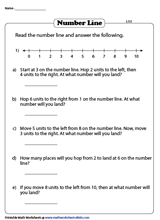Become a Member

Membership Information

What's New?

Printing Help

Testimonial## Number LineVisualize and work with numbers in sequence on a virtual number line with or without tick marks.## App Tutorial

The Number Line app helps students visualize number sequences and illustrate strategies for counting, comparing, adding, subtracting, multiplying, and dividing. Choose number lines labeled with whole numbers, fractions, decimals, or negative numbers. Or use a custom number line, with or without tick marks, and set your own interval.

Show intervals between points on the line using forward or backward jumps above and below the line. Jumps can also be labeled with their values or left blank. Add custom tick marks to the number line to show equivalence or compare number values.

The Number Line app is an open-ended educational tool, ideal for elementary classrooms and other learning environments that use laptops, iPads, or Chromebooks.

The number line is a key model used in Bridges in Mathematics , second edition.  Online preview available .

## App Features

• Set up a number line with whole number, fraction, or decimal intervals.
• Add and manipulate forward and backward jumps, above or below the line.
• Label jumps, manually or automatically, to show intervals or hide labels altogether.
• Add custom tick marks and values to open number lines.
• Share your work by saving an image or creating an 8-character code and link to send to others.

## Related Products

• Number Line Pocket Chart
• Number Line Display Cards

## Get More From This App

Book for educators.The number line is a common artifact in K–5 classrooms but is seldom used to its potential. This book is filled with theoretical and practical information that can make the number line a powerful model for mathematical learning. Teachers learn how to implement lessons that help grow students’ confidence, fluency, and facility with numbers. Engaging activities support students’ development of intuitive strategies for single- and multiple-digit addition and subtraction.

## Acknowledgements

Number Line was made possible by a generous donation from Dr. David Moursund.

## Technical Support and Feedback

© 2022 The Math Learning Center

This app was crafted by Clarity Innovations: “We improve teaching and learning by creating solutions that match promising technologies with the needs of education.” www.clarity-innovations.com .

Number Line• Microsoft Teams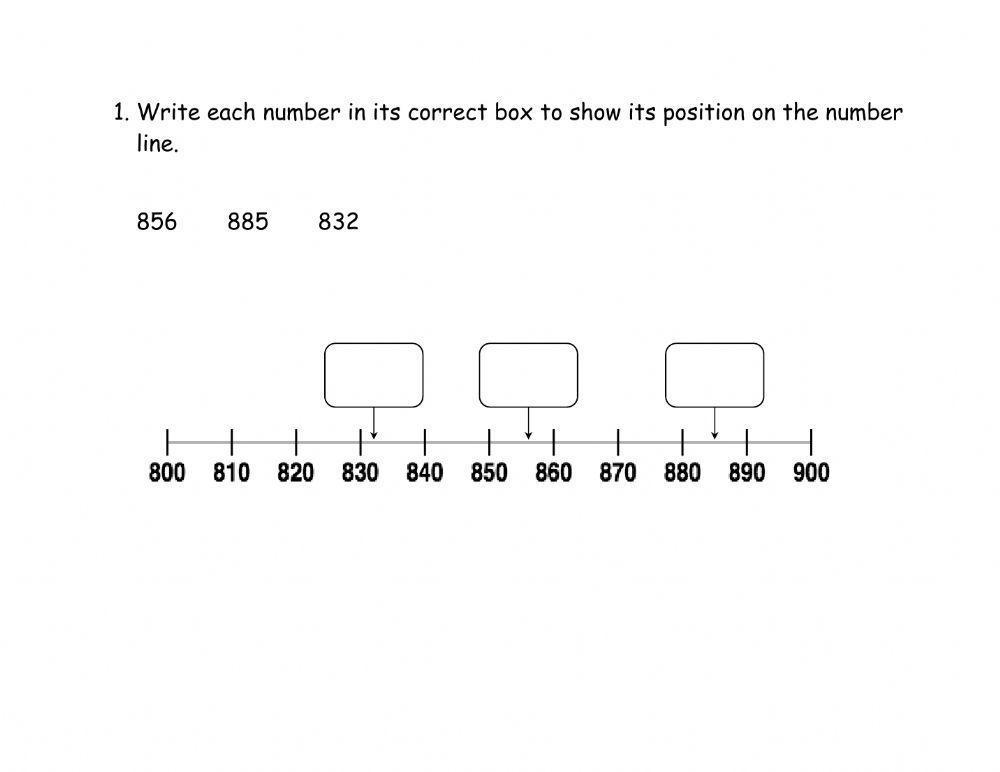Algebra & Pre-Algebra

Comparing Numbers

Daily Math Review

Division (Basic)

Division (Long Division)

Hundreds Charts

Measurement

Multiplication (Basic)

Multiplication (Multi-Digit)

Order of Operations

Place Value

Probability

Skip Counting

Subtraction

Telling Time

Word Problems (Daily)

More Math Worksheets

Cause & Effect

Fact & Opinion

Fix the Sentences

Graphic Organizers

Synonyms & Antonyms

Writing Prompts

Writing Story Pictures

Writing Worksheets

More ELA Worksheets

Consonant Sounds

Vowel Sounds

Consonant Blends

Consonant Digraphs

Word Families

More Phonics Worksheets

## Early Literacy

Build Sentences

Sight Word Units

Sight Words (Individual)

More Early Literacy

Punctuation

Subjects and Predicates

More Grammar Worksheets

## Spelling Lists

More Spelling Worksheets

## Chapter Books

Charlotte's Web

Magic Tree House #1

Boxcar Children

More Literacy Units

Animal (Vertebrate) Groups

Animal Articles

Butterfly Life Cycle

Electricity

Matter (Solid, Liquid, Gas)

Simple Machines

Space - Solar System

More Science Worksheets

## Social Studies

Maps (Geography)

Maps (Map Skills)

More Social Studies

Back-to-School

Autumn Worksheets

Halloween Worksheets

Christmas Worksheets

More Holiday Worksheets

## Puzzles & Brain Teasers

Brain Teasers

Mystery Graph Pictures

Number Detective

Lost in the USA

More Thinking Puzzles

## Teacher Helpers

Teaching Tools

Award Certificates

More Teacher Helpers

## Pre-K and Kindergarten

Alphabet (ABCs)

Numbers and Counting

Shapes (Basic)

More Kindergarten

## Worksheet Generator

Word Search Generator

Multiple Choice Generator

Fill-in-the-Blanks Generator

More Generator Tools

Full Website Index

## Number Line Worksheets

We have printable number line worksheets for teaching counting, skip counting, addition, subtraction, and number patterns.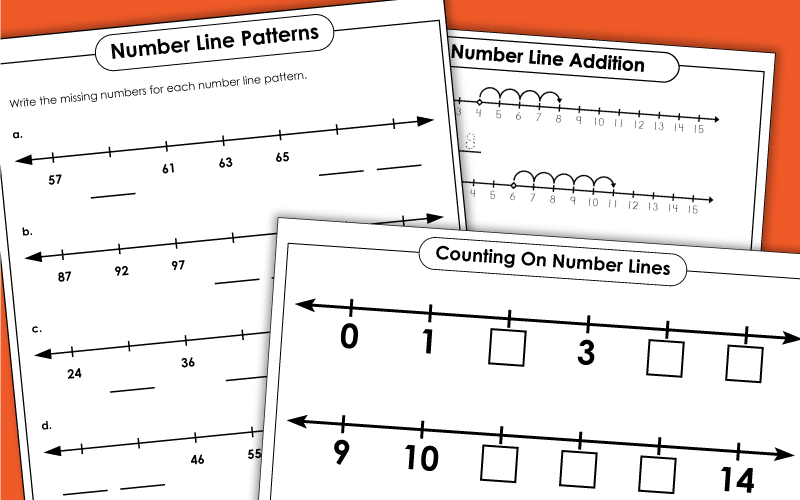## Number LinesLogged in members can use the Super Teacher Worksheets filing cabinet to save their favorite worksheets.## Counting Number Lines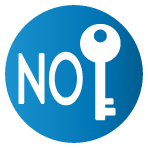Number line subtraction, skip counting with number lines.## Number Line Patterns

Fractions and decimal number lines, double number lines fractions, decimals, percents.

Super Teacher Worksheets has a huge collection of math worksheets for most skills taught in the elementary grades.

Complete the number patterns and write the rule for each.

Practice skip counting by 2s, 3s, 4s, 5s, 10s, 25s, and 100s.

Hundreds charts can also be used to teach place value, adding, subtracting, skip counting, and number patterns.

## Pictures of Our Worksheets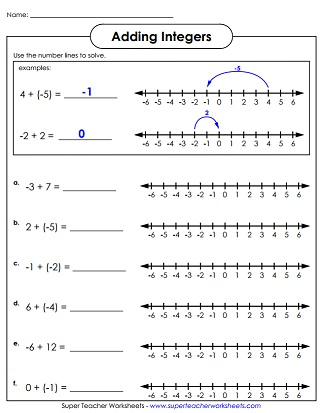• Home   |
• Privacy   |
• Shop   |
• 🔍 Search Site
• Halloween Color By Number
• Halloween Dot to Dot
• Kindergarten Halloween Sheets
• Puzzles & Challenges for Older Kids
• Christmas Worksheets
• Easter Color By Number Sheets
• Printable Easter Dot to Dot
• Easter Worksheets for kids
• Kindergarten
• All Generated Sheets
• Place Value Generated Sheets
• Subtraction Generated Sheets
• Multiplication Generated Sheets
• Division Generated Sheets
• Money Generated Sheets
• Negative Numbers Generated Sheets
• Fraction Generated Sheets
• Place Value Zones
• Number Bonds
• Times Tables
• Fraction & Percent Zones
• All Calculators
• Fraction Calculators
• Percent calculators
• Area & Volume Calculators
• Age Calculator
• Height Calculator
• Roman Numeral Calculator
• Coloring Pages
• Fun Math Sheets
• Math Puzzles
• Mental Math Sheets
• Online Times Tables
• Math Grab Packs
• All Math Quizzes
• Place Value
• Rounding Numbers
• Comparing Numbers
• Number Lines
• Prime Numbers
• Negative Numbers
• Roman Numerals
• Subtraction
• Multiplication
• Fraction Worksheets
• Learning Fractions
• Fraction Printables
• Percent Worksheets & Help
• All Geometry
• 2d Shapes Worksheets
• 3d Shapes Worksheets
• Shape Properties
• Geometry Cheat Sheets
• Printable Shapes
• Coordinates
• Measurement
• Math Conversion
• Statistics Worksheets
• Bar Graph Worksheets
• Venn Diagrams
• All Word Problems
• Finding all possibilities
• Logic Problems
• Ratio Word Problems
• All UK Maths Sheets
• Year 1 Maths Worksheets
• Year 2 Maths Worksheets
• Year 3 Maths Worksheets
• Year 4 Maths Worksheets
• Year 5 Maths Worksheets
• Year 6 Maths Worksheets
• All AU Maths Sheets
• Kindergarten Maths Australia
• Year 1 Maths Australia
• Year 2 Maths Australia
• Year 3 Maths Australia
• Year 4 Maths Australia
• Year 5 Maths Australia
• Meet the Sallies
• Certificates

## Number Line Worksheets up to 1000

Welcome to the Math Salamanders Number Line Worksheets collection.

Here you will find a wide range of free printable number line sheets, which will help your child understand how to count on and back in 1s, 2s, 5s, 10s, 50s and 100s.

The sheets will also help with your child's understanding of place value to 1000.

For full functionality of this site it is necessary to enable JavaScript.

Number line worksheets to 200, number line worksheets to 1000.

• Number Line up to 1000 Online Quiz
• More related Math resources

## Number Line Worksheets for 2nd Grade

Here you will find a selection of 2nd Grade Math worksheets designed to help your child understand how to position numbers up to 1000 correctly on a number line. The sheets are graded so that the easier ones are at the top.

The harder sheets at the end of each section will help to extend more able mathematicians.

Each sheets comes with an answer sheet for quick reference.

• count on and back in 1s 2s 5s 10s 50s and 100s;
• place a number correctly on a number line up to 1000.
• Number Line to 200 Sheet 1
• Number Line to 200 Sheet 2
• Number Line to 200 Sheet 3
• Number Line to 200 Sheet 4
• Number Line to 200 Sheet 5
• Number Line to 1000 Sheet 1
• Number Line to 1000 Sheet 2
• Number Line to 1000 Sheet 3
• Number Line to 1000 Sheet 4
• Number Line to 1000 Sheet 5
• Number Line to 1000 Sheet 6 (Estimation)

## More Recommended Math Worksheets

Take a look at some more of our worksheets similar to these.

## 2nd Grade Place Value Worksheets

Below are our selection of Place Value, Ordering and Rounding Worksheets

The following worksheets involve different Second Grade place value activities such as counting in hundreds, tens and ones, reading, writing and ordering numbers to 1000, and know what number each digit represents.

• learn to count by hundreds, tens, fives, twos and ones;
• learn their place value to 1000;
• order numbers to 1000.

All the free 2nd Grade Math practice sheets in this section are informed by the Elementary Math Benchmarks for Second Grade.

• Second Grade Place Value Worksheets to 1000
• 2nd Grade Counting Worksheets 1s 2s 5s 10s 100s

## Ordering and Rounding Worksheets to 1000

Once your child is confident with their place value to 1000, they can start learning about ordering and rounding numbers.

• Ordering Numbers Worksheets to 1000
• Rounding to the nearest 10 Worksheets

Here is our selection of measurement worksheets for 2nd graders.

These sheets involve reading scales going up in ones, fives, tens and hundreds to find the length, weight or liquid capacity.

Using these sheets will help children to consolidate their counting as well as learning to read a simple scale.

## Number Lines up to 1000 Quiz

Our quizzes have been created using Google Forms.

At the end of the quiz, you will get the chance to see your results by clicking 'See Score'.

This will take you to a new webpage where your results will be shown. You can print a copy of your results from this page, either as a pdf or as a paper copy.

For incorrect responses, we have added some helpful learning points to explain which answer was correct and why.

We do not collect any personal data from our quizzes, except in the 'First Name' and 'Group/Class' fields which are both optional and only used for teachers to identify students within their educational setting.

We also collect the results from the quizzes which we use to help us to develop our resources and give us insight into future resources to create.

This quick quiz tests your understanding and skill at positioning and reading numbers on number lines from 0 to 1000.

How to Print or Save these sheetsNeed help with printing or saving? Follow these 3 steps to get your worksheets printed perfectly!

• How to Print support

## Math-Salamanders.com

The Math Salamanders hope you enjoy using these free printable Math worksheets and all our other Math games and resources.

TOP OF PAGE• Puzzles & Challenges

## Placing Numbers on Number Lines from 0 to 50 (A)

Welcome to The Placing Numbers on Number Lines from 0 to 50 (A) Math Worksheet from the Number Line Worksheets Page at Math-Drills.com. This math worksheet was created or last revised on 2022-11-30 and has been viewed 22 times this week and 58 times this month. It may be printed, downloaded or saved and used in your classroom, home school, or other educational environment to help someone learn math.

Teacher s can use math worksheets as test s, practice assignment s or teaching tool s (for example in group work , for scaffolding or in a learning center ). Parent s can work with their children to give them extra practice , to help them learn a new math skill or to keep their skills fresh over school breaks . Student s can use math worksheets to master a math skill through practice, in a study group or for peer tutoring .

Use the buttons below to print, open, or download the PDF version of the Placing Numbers on Number Lines from 0 to 50 (A) math worksheet . The size of the PDF file is 14975 bytes . Preview images of the first and second (if there is one) pages are shown. If there are more versions of this worksheet, the other versions will be available below the preview images. For more like this, use the search bar to look for some or all of these keywords: mathematics, placement, placing, locating, location, numberlines, number, lines, sense, numeracy .

Print Full Version

Open Full Version

Print Student Version

Open Student Version

The Print button initiates your browser's print dialog. The Open button opens the complete PDF file in a new browser tab. The Download button initiates a download of the PDF math worksheet. Teacher versions include both the question page and the answer key. Student versions, if present, include only the question page.Other Versions:

More Number Line Worksheets

## Number Line Worksheets

Number line worksheets can be used as a starting point to practice problems based on number lines. These worksheets include questions on plotting numbers on the number line, word problems, interpretive problems and so on.

## Benefits of Number Line Worksheets

Number line worksheets can act as an aid in helping students to visualize certain operations such as addition and subtraction. By solving multiple problems on the topic, students will inevitably master the concept and apply it to problems sums with ease.

The problems are arranged in an increasing order of difficulty to ensure that a student proceeds from one level to another only after he has cleared his basic concepts. They also provide immense flexibility so that a student does not feel pressured while studying.

The worksheets can be used as a guide in preparing for competitive exams such as Olympiads. They are easy to use, free to download, and available in the form of PDFs.

## ☛ Check Grade wise Number Line Worksheets

• Kindergarten Number Line Worksheets
• 1st Grade Number Line Worksheets
• Number Line Worksheets for Grade 2
• 3rd Grade Number Line Worksheets

## Number Line WorksheetsNumber line worksheets make learning basic addition and subtraction facts much easier for the new math student.

They give students a way to visualize the math problem, making an abstract concept concrete.  If you've never used a number line, they're simple to use.

If your child has an addition problem, for example 5+3, he or she can place a finger on the first number on the number line, in this case the number 5.  Then the student simply moves the finger three more spots on the number line and lands on the correct answer, in this case, it would be 8.

In addition to the worksheets, I've also created a few printable number lines that you can use along with the practice sheets found in my 'Fractions' and 'Decimals' lessons.  You can find those worksheets at the bottom of the page. Here are a few examples of the worksheets you'll find in the lessons below.## Number Line Worksheets & Activities

Click on any image below to print out your number line or worksheets.

The first 3 units link to printable number lines covering decimals, fractions, and numbers.  They would be great tools to use as your students learn about those concepts.  I've created many worksheets covering those topics which you can find using the menu.

I've made multiple variations of the Printable Number Line that you can print out.  Beginner mathematicians should start with the numbers 1-10.  There is also a line that goes to twenty and one that shows both positive and negative numbers. As for the addition worksheets, there are lessons that focus solely on addition or subtraction and ones that combine the two.  You can also print out some blank templates to create your own math problems.

After you've printed out the decimal and fraction lines, you can use that as a guide in the lessons below.More Sample Papers:Try These Out Next:• New Worksheets
• Connect the Dots
• Flashcards (Printable)
• Math Charts & Tables
• Multiplication
• Multiplication Tables
• Number Lines
• Place Value
• Skip Counting
• Coloring Pages
• Writing Prompts
• Consonant Letters
• Short Vowels
• Sight Words
• Valentine's Day
• St. Patrick's Day
• Mother's Day
• Thanksgiving

• KindergartenWe don't have your cell phone number on file.

With a cell phone number on file, we can provide an alternate way to contact you in case you need to reset your password. Click here. to edit your account information and add a phone number.

Number Problems Money Story Problems BASIS CTE Change Views Search Custom Headers

While you are here, as a way of helping me to get to know my users, would you be kind enough take a couple of minutes to fill out this survey? Thank you!

Find the equation of a line from 2 points. Find the equation of a line that is perpendicular to a given line and runs through a given point. Find the equation of a line that is parallel to a given line and runs through a given point. Find the equation of a line from the Slope and One Point.

The Math Worksheet Site is provided by Scott Bryce . [email protected]

For tech support: [email protected] . For customer service: [email protected]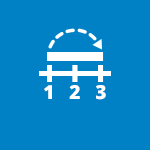## Number Line, by the Math Learning Center

Number line helps students visualize number sequences and illustrate strategies for counting, comparing, adding, subtracting, multiplying, and dividing whole numbers. the number line can be labeled with multiples of any whole number from 1 to 100. or select a blank number line—with or without tick marks—and place custom tick marks anywhere on the line with the option of entering a custom number label. hide elements of the sequence shown on the number line, which challenges students to use what they know about number relationships and computation to identify those missing elements. place forward or backward jumps above or below the line to show number intervals or differences. jumps can also be labelled with their values or left blank. this virtual number line is an open-ended educational tool, ideal for elementary classrooms. for more number line resources and other apps, visit: www.mathlearningcenter.org/apps, 11/3/2015 7:46:16 am.#### IMAGES

1. Number Line Worksheets pdf printable2. FREE Printable Number Line Worksheet3. Comparing Numbers With Number Lines Worksheets4. Number Line 1005. Free Math Worksheets Number Lines6. Kindergarten Math Printables#### VIDEO

1. Math Worksheet || school Activities || ભારે વસ્તુ અને હલકી વસ્તુ #youtubeshorts #math #youtube

2. Understand addition Using Number Lines

3. Addition of numbers on number line संख्या रेखा

4. Class 9 Maths || How to represent √3 on number line || Let's Start Learning

5. My Math project. On number line

6. Number Lines Tricks 📚 #Shorts #maths #mathematics #education #learn#tricks #class8 #class9 #class10

1. The Math Worksheet Site.com -- Number Lines

Number Lines Values may be whole numbers, negative numbers or decimals. In order to provide flexibility in creating number lines, this site does not limit the values you may use to create number lines. The generator will attempt to create a number line with whatever data is provided. Not all values will create useful number lines. Starting Number

2. The Math Worksheet Site.com

The Math Worksheet site has highly customizable, generated worksheets that target your students' specific needs. Whether you are teaching kindergartens how to count, youngsters how to multiply, teens how to factor polynomials, or adults how to understand Ohm's law, you will find what you need at The Math Worksheet Site.

3. Number Line Worksheets

This page includes Number line worksheets for learning about the ordinality of numbers and for use as a math aid. Most Popular Number Line Worksheets this Week Adding and Subtracting up to 100 on Number Lines with Intervals of 10 ( 536 views this week) Number Lines from 1 to 25 Counting by 1 ( 315 views this week)

4. Number Line, by The Math Learning Center

Number Line helps students visualize number sequences and demonstrate strategies for counting, comparing, adding, subtracting, multiplying, and dividing.

5. The Math Worksheet Site.com -- Walking the Number Line

The Math Worksheet Site.com -- Walking the Number Line Walking the Number Line Walking the number line is a concept used to help kids understand adding and subtracting negative numbers. A number line can be drawn on the floor, or a small plastic figure can be used to walk the number line on paper.

6. Number Line Worksheets

These worksheets include an assortment of topics like identifying whole numbers, integers, fractions and decimals using number lines; addition, subtraction, multiplication and division using number lines and more! List of Number Line Worksheets Number line templates Identify the whole numbers Addition using a number line

7. The Math Worksheet Site.com -- Mixed Numbers: Number Lines

Mixed Numbers on Number Lines Use positive numbers only. Use negative numbers only. Use both positive and negative numbers. The student will mark the point for the given number. The student write the number for the given point. Number of divisions will always match the denominator.

8. The Math Worksheet Site.com -- Subtraction: Number Lines

The Math Worksheet Site.com -- Subtraction: Number Lines Subtraction: Number Lines Number Lines 0 through 10 0 through 15 -- subtract numbers less than 10 0 through 15 -- subtract numbers up to 14 0 through 20 -- subtract numbers less than 10 0 through 20 -- subtract numbers up to 19 -10 through 10 -- subtract numbers from -10 to 10 Problem Type

9. Number Line

The number line is a common artifact in K-5 classrooms but is seldom used to its potential. This book is filled with theoretical and practical information that can make the number line a powerful model for mathematical learning. Teachers learn how to implement lessons that help grow students' confidence, fluency, and facility with numbers ...

10. Number Line interactive worksheet

Liveworksheets transforms your traditional printable worksheets into self-correcting interactive exercises that the students can do online and send to the teacher. ... Math (1061955) Main content: Number Line (2026787) Number Line. Other contents: number line Share / Print Worksheet. Google Classroom Microsoft Teams Facebook Pinterest

11. Number Line Worksheets

These Number Line Worksheets will produce a page of horizontal number lines for various types of scales, start points, end points and increments. These Number Line Worksheets are appropriate for Kindergarten, 1st Grade, 2nd Grade, 3rd Grade, 4th Grade, 5th Grade, and 6th Grade. Vertical Number Line Graphing Paper Worksheets

12. Math Number Line Sheets

Math Number Line Sheets | | Math Number Line Hub Page Welcome to the Math Salamanders Math Number Lines. Here you will find a wide range of free printable number lines, which will help your child to understand place value and order numbers.

13. Number Line Worksheets

We have printable number line worksheets for teaching counting, skip counting, addition, subtraction, and number patterns. Number Lines Number Line 1-10 - Cut & Glue FREE Cut out the parts of this number line and glue them together. This number line includes numbers one through ten. Kindergarten and 1st Grade View PDF Basic 0-20 Number Line (Small)

14. Browse Printable Math Worksheets

1,939 filtered results Math number line Show interactive only Sort by Number Line Interactive Worksheet Fill in the Number Line Worksheet Subtraction Number Line Worksheet Addition Using a Number Line Worksheet Subtraction Across the Number Line Worksheet Number Line Addition Worksheet Number Line 1-20 Worksheet Adding With a Number Line

15. Number Lines

Number Lines Create Number Lines Worksheets! Values may be whole numbers, negative numbers or decimals. Fill out the form below and click " Create It ". To create a new worksheet, just click "Refresh Page" button below. To download or print the worksheet, just click the icon in the upper right hand corner of the worksheet viewer

16. Number Line Worksheets up to 1000

up to 1000. Welcome to the Math Salamanders Number Line Worksheets collection. Here you will find a wide range of free printable number line sheets, which will help your child understand how to count on and back in 1s, 2s, 5s, 10s, 50s and 100s. The sheets will also help with your child's understanding of place value to 1000.

17. Number Line Worksheets

Number Line Graph Paper Worksheets. This Number Line Worksheet will produce a blank page of Number Lines for various types of scales. You may enter whole numbers, negative numbers or decimals numbers for the starting and ending numbers. You may select increments that are whole integers or fractions.

18. Free Number Line Worksheet Generator

Number Line Math worksheet generator Options Theme. Enter number line content Customize the range and increments for your number line. See help: help. Define the range for your number line by entering a starting number and an ending number. If you'd like to use an increment value greater than 1, you have the option of entering a custom value in ...

19. Placing Numbers on Number Lines from 0 to 50 (A)

Students can use math worksheets to master a math skill through practice, in a study group or for peer tutoring. Use the buttons below to print, open, or download the PDF version of the Placing Numbers on Number Lines from 0 to 50 (A) math worksheet. The size of the PDF file is 14975 bytes. Preview images of the first and second (if there is ...

20. Number Line Worksheets

Number line worksheets can be used as a starting point to practice problems based on number lines. These worksheets include questions on plotting numbers on the number line, word problems, interpretive problems and so on. Benefits of Number Line Worksheets

21. Number Line Worksheets

Number line worksheets make learning basic addition and subtraction facts much easier for the new math student. They give students a way to visualize the math problem, making an abstract concept concrete. If you've never used a number line, they're simple to use. If your child has an addition problem, for example 5+3, he or she can place a ...

22. The Math Worksheet Site.com

Number Recognition Which is X? How Many? Count Scattered Objects Fill in the Missing Numbers Ordinal Numbers Ordinals - Secret Word Skip Counting Odd or Even Odd or Even (Balloons) Tally Marks Ten Frames Counting Tree Rings Time Clock Faces Telling Time Telling Time: Matching Adding and Subtracting Time Add and Subtract Time (2)

23. Number Line, by the Math Learning Center

11/3/2015 7:46:16 AM. Number Line helps students visualize number sequences and illustrate strategies for counting, comparing, adding, subtracting, multiplying, and dividing whole numbers. The number line can be labeled with multiples of any whole number from 1 to 100. Or select a blank number line—with or without tick marks—and place ...